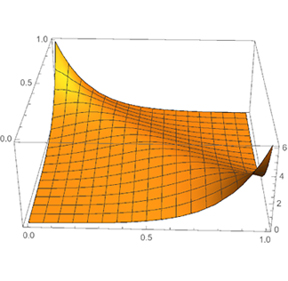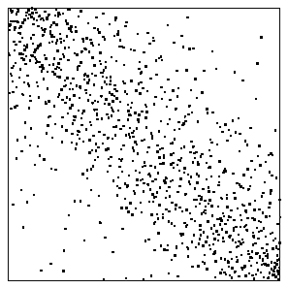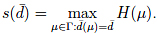### Highlights#### Limits of Permutations

ICERM - October 2015In a well-shuffled deck of cards, about half of the pairs of cards are out of order. Mathematically, we say that in a permutation $\pi$ of $[n]=\{1,2,\dots,n\}$ there are about $\frac12\binom{n}{2}$ inversions, that is, pairs $i<j$ for which $\pi(j)<\pi(i)$.  Suppose we are interested in studying permutations for which the number of inversions is exceptionally large or small, for example $\alpha\binom{n}{2}$ for some other $\alpha\in[0,1]$. What can one say about the structure and properties of such a permutation? For example, typically how many double inversions (triples $i<j<k$ for which $\pi(k)<\pi(j)<\pi(i)$) might one expect?Figure 1: A permuton with density $3/4$ of inversions.

Figure 1 is an illustration of the shape of a large permutation with a fraction $3/4$ of inversions ($\frac34\binom{n}{2}$ total inversions), in a large-$n$ limit. What we are actually drawing is called a permuton: a probability measure on $[0,1]^2$ with uniform marginals (well, we’re graphing its probability density function). How does a permuton describe a large permutation? A permuton is, in a well-defined sense, the limit of the scaled permutation matrices associated to larger and larger permutations. The permutation matrix associated to a permutation of $[n=300]$ and $3/4$ fraction of inversions is shown in Figure 2.Figure 2: A permutation matrix of a uniform random permutation with density $3/4$ of inversions.

Generally a pattern in a permutation is an order-sequence imposed on a finite subset of $[n]$; for instance, the permutation 34251 contains 5 copies of the pattern 231 (342, 341, 351, 451, and 251).  The density of a pattern $\pi$ of length $k$ in a permutation $\sigma$ on $[n]$ is the number of occurrences of the pattern $\pi$, divided by $n \choose k$.

If we fix the densities $d_1,d_2 \ldots$ of a few specific patterns, such as 12 and 123, the set of all permutations in $S_n$ with approximately those densities takes on a bulk persona’, or limit shape. Most permutations in the set look alike; for example, their other pattern densities match.  Moreover, the limit shape, with
these constraints, varies smoothly (in fact analytically) except for an occasional singularity when a phase boundary’ is crossed. This is why we can assert that
almost all permutations with density $3/4$ of inversions look like’ Figure 1.

How do we find the permuton for a given set of densities? This can be realized with the recent derivation [Kenyon, Král’, Radin, Winkler: arxiv:1506.02340] of a variational principle. Starting from the fact that the number of permutations in $S_n$ with given constraints $\bar%20d$ is generally of the order $n!\exp(sn)$, the (negative) entropy’ $s=s(\bar%20d)$, maximizing entropy allows one to find the permuton. That is, one can define an entropy function $H$ on the space $\Gamma$ of permutons and the desired permuton is obtained by maximizing $H$ over all permutons satisfying the given pattern constraints $\bar%20d$:This gives a way to understand what a typical large, constrained permutation is like. If, as seems to be the usual case, there is a unique optimal permuton $\tilde \mu$ representing $s(\bar%20d)$, we can, via integration over $\tilde\mu$, obtain all other pattern densities of a typical large permutation with constraints $\bar%20d$. This opens up a world of phases and phase transitions in a new setting.

This variational principle was an outgrowth of the Spring 2015 Phase Transitions and Emergent Properties program of the Institute for Computational and Experimental Research in Mathematics (ICERM).